Skip to main content

Volume 7 Supplement 5

# Asymptotic behaviour and optimal word size for exact and approximate word matches between random sequences

## Abstract

### Background

The number of k-words shared between two sequences is a simple and effcient alignment-free sequence comparison method. This statistic, D2, has been used for the clustering of EST sequences. Sequence comparison based on D2 is extremely fast, its runtime is proportional to the size of the sequences under scrutiny, whereas alignment-based comparisons have a worst-case run time proportional to the square of the size. Recent studies have tackled the rigorous study of the statistical distribution of D2, and asymptotic regimes have been derived. The distribution of approximate k-word matches has also been studied.

### Results

We have computed the D2 optimal word size for various sequence lengths, and for both perfect and approximate word matches. Kolmogorov-Smirnov tests show D2 to have a compound Poisson distribution at the optimal word size for small sequence lengths (below 400 letters) and a normal distribution at the optimal word size for large sequence lengths (above 1600 letters). We find that the D2 statistic outperforms BLAST in the comparison of artificially evolved sequences, and performs similarly to other methods based on exact word matches. These results obtained with randomly generated sequences are also valid for sequences derived from human genomic DNA.

### Conclusion

We have characterized the distribution of the D2 statistic at optimal word sizes. We find that the best trade-off between computational efficiency and accuracy is obtained with exact word matches. Given that our numerical tests have not included sequence shuffling, transposition or splicing, the improvements over existing methods reported here underestimate that expected in real sequences. Because of the linear run time and of the known normal asymptotic behavior, D2-based methods are most appropriate for large genomic sequences.

## Background

The overwhelming amount of molecular data generated by the sequencing of whole genomes and EST libraries has triggered the development of numerous sequence comparison algorithms, aimed at detecting related sequences and at quantifying this relatedness. BLAST , FASTA  and other related algorithm are arguably the most popular programs for sequence comparison. These algorithms rely on the local alignment of the sequences under scrutiny and assume conservation of contiguity between homologous segments. This assumption, however, is often violated. Discontinuity can occur, for example, when spliced transcripts are matched to genomic sequences, when ESTs or cDNAs from different splice variants are compared or when genomic sequences are aligned that have undergone genome shuffling. Other alignment-based algorithms that do not assume conservation of contiguity, such as BLAT  or SIM4 , can compute scores, percentage similarity, but do not assess statistical significance.

Several types of alignment-free sequence comparison algorithms, reviewed in , can circumvent this problem. Among these alignment-free methods, techniques based on the number of k-words shared between two sequences, also known as the D2 statistic, are particularly noteworthy due to the speed of their implementation, their sensitivity and selectivity . They have been extensively used to structure large collections of ESTs into clusters of similar sequences .

The rigorous study of the statistical distribution of D2 began with the computation of bounds of D2 variance and the characterization of asymptotic distributional regimes . These results have been refined in a recent study . Other studies [12, 13] have focussed on a generalization of D2, the number of approximate k-word matches between two sequences,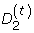, where t is the number of mismatches per word. Bounds on thevariance and asymptotic distribution ofhave been determined .

The current paper summarizes the theoretical knowledge on the distribution of D2 and. The optimal word sizes of these statistics in a variety of conditions were computed, and the distributional regimes at optimal word size were deduced. Finally, the accuracy ofas a measure of sequence similarity was compared with other measures using random sequences and sequences evolved from human genomic DNA.

## Results and Discussion

### Word matches measures

#### Exact matches

The theory developed for the number of exact word matches between two sequences is widely applicable to any kind of sequence with the only constraint that these are made of independent and identically distributed (i.i.d.) letters. Given an alphabetof d letters, let A = A1A2 A n be a sequence of n i.i.d. letters of. Let f a be the probability of a letter taking the value a, and p k =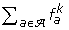.

D2 is defined as the number of k-words matches between two sequences A and B, and can be expressed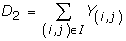where Y(i, j)is the k-word match indicator variable starting at position i in A and j in B, and the index set is

I = {(i, j) : 1 ≤ i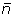, 1 ≤ j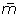}

where, for convenience, (n - k + 1) and (m - k + 1) are writtenandrespectively.

D2 can also be thought of as an inner product of the word count vectors. Let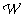= {w 1 , w 2 , , w n } be the set of all k-words on. For w, let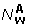be the number of times the letter w appears in sequence A. The count vector for that sequence is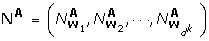. D2 can thus also be expressed as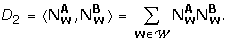The mean of D2 can be easily computed (e.g. )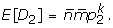When the letters of the alphabet are uniformly distributed, that is, f a =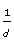, for all a A.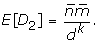Lower and upper bounds for the variance of D2 were computed in , and an exact formula for the variance in the case of uniform letter distribution was given in . Limiting distributions of D2 when n and k get large were derived in , namely (a) when k/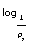n > 2, and E[D2] is not too small, D2 has a compound Poisson  asymptotic behaviour, and (b) when k/n < 1/6, and the letter distribution is non-uniform, D2 has a normal asymptotic behaviour. Numerical simulations in  showed that these theoretical bounds are not tight. The uniform letter distribution case was studied in , where it was proved that, for large enough k, the D2 statistic is approximately normal as n gets large.

#### Approximate matches

The theory for the number of approximate matches between two sequences has been developed in the more restricted framework of strand symmetric Bernoulli text [12, 13]. In this framework, the letters are i.i.d. with frequencies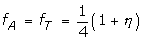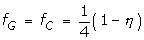where η is the perturbation parameter, with -1 ≥ η ≥ 1. The distance between two words x and y of length k is defined as the number of character mismatches between x and y and is written δ(x, y). When δ(x, y) ≥ t, x is said to be a t-neighbour of y. If A is a strand symmetric Bernoulli text and w is a known word of the same length, n, as A, then the probability distribution of the distance δ(A, w) is:

Pr(δ(A, w) = t) =g t (n,η,c)

where g t is a perturbed binomial distribution , c is the GC count in w and η is the perturbation parameter of A. The cumulative distribution function of the distance is then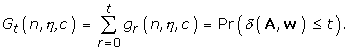Thestatistic is defined to be the number of t-neighbours of k-words between sequences A and B, and can be expressed as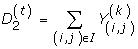where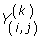is the indicator variable of t-neighbourhood for the k-words starting at position i in A and j in B. The expectation ofcan be expressed in terms of the perturbed binomial distribution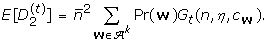Upper and lower bounds for the variance ofwere computed in , and the following limit deduced: for large n,is asymptotically normal when k = α(n) + C where 0 ≥ α <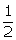and C is a constant. When t = 0, this result is an improvement on the α = 1/6 result for perfect matches  reported above. Numerical simulations in  suggest that this asymptotic behaviour occurs for α as high as 2.

The results of the numerical simulations comparing the distribution ofto a normal distribution, for various word sizes, sequences sizes and number of mismatches, in the case of non-uniform letters distribution, are shown in figure 1. Similar tables comparing D2 to the normal and compound Poisson distribution, in the case of exact word matches, for uniform and non-uniform letters distribution, can be found in .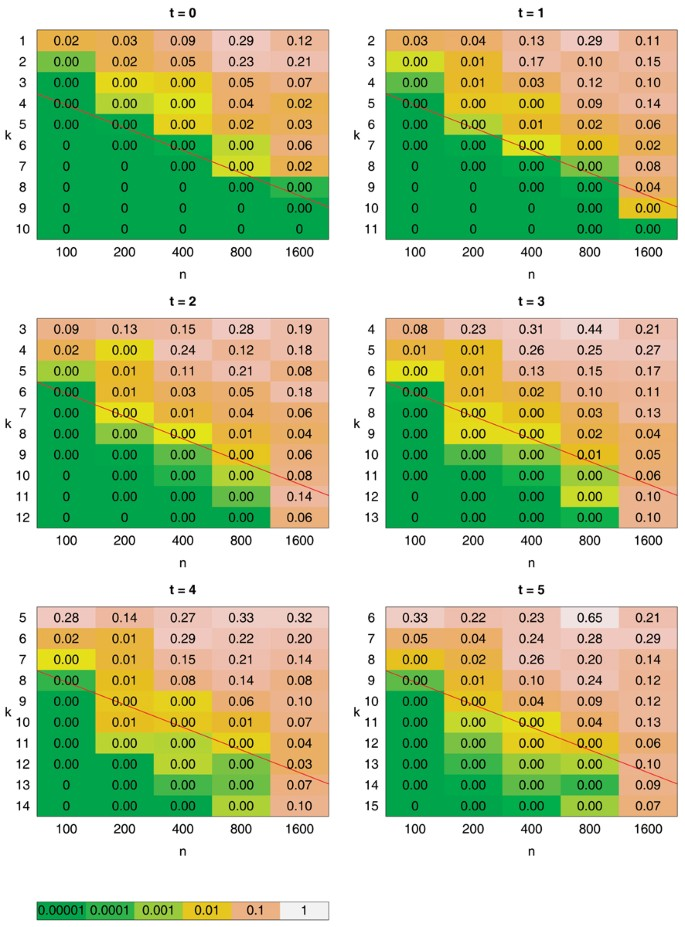Figure 1

### Optimal word sizes

Our first goal is to characterize the distribution regime ofwhen the word size is optimal. We write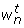the optimal word size for sequences of size n and t mismatches. Numerical simulations were carried out to determinefor various sequence lengths n = 2x × 102 with x = 1, 2, 3, 4, 5, and for different numbers of mismatches t = 0, 1, 2, 3, 4, 5. A summary of these simulations is given in table 1 and the detailed simulation results can be found in additional files 1 to 9. Sequences with a non-uniform letter distribution were used, with nucleotide frequencies f A = f T =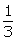, f G = f C =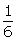. Similar compositional biases are observed in several sequenced genomes, such as the honey bee Apis mellifera, the roundworm Caenorhabditis elegans or the zebra fish Danio rerio. The optimal word size was also computed in the uniform case for t = 0, 3, with very similar outcomes. We will therefore focus our discussion on the non-uniform case.

Regardless of the number of mismatches, the optimal word size is quite stable when the lengths of the sequences vary across the range of sizes under consideration. We only noticed a slight decrease infor sequences smaller than 400 letters (see table 1). The behaviour of, however, can traverse different distribution regimes when the sequence length varies and the word size is fixed.

When our data for perfect word matches are compared to tables 1 and 3 in , it appears that the distribution of D2 at optimal word size is approximately a compound Poisson distribution when sequences are 400 letters long or smaller, and is approximately normal when sequences lengths are larger than 1600 letters.

In the case of approximate word matches, it can be extrapolated from figure 1 that the distribution ofbecomes normal for sequences larger than 1600 letters. The approximate behaviour of, when t > 0 and k/n > 2, however, is unknown. The distribution regime ofat optimal word size for smaller sequences could therefore not be characterized.

### Accuracy ofmeasures

A previous study  compared the accuracy of various dissimilarity measures based on the number of words shared between 2 sequences. These authors give the values of the Spearman's rank statistic, A (see methods), obtained with these measures and compare them with that obtained using either BLAST or the Hamming distance. Similarly, we compared the efficiency offor different numbers of mismatches, t = 0,1, 2, 3, 4, 5 (figure 2). We used the same sequence size (600) as inthe above-cited study so as to allow comparison of the performance ofwith the measures assessed in that study. A for BLAST was computed based on the bit scores obtained with the default settings.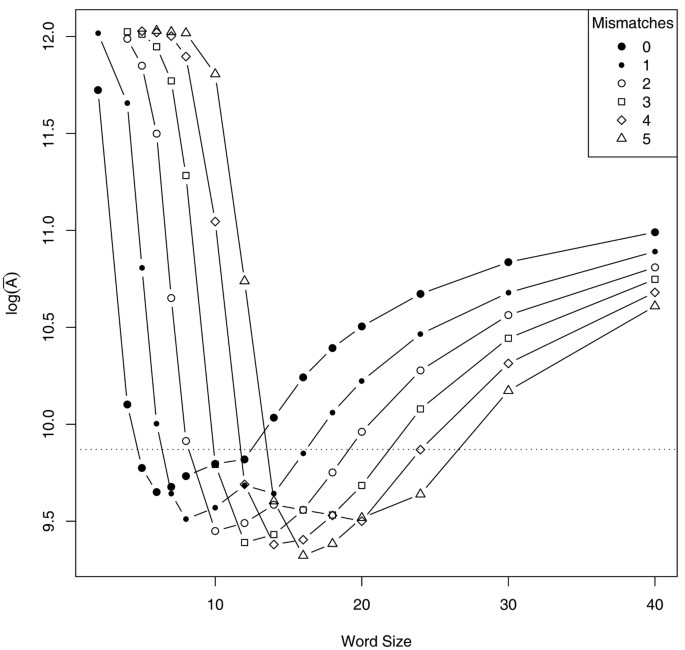Figure 2

Overall, themeasures provide an accuracy similar to the dissimilarity measures computed in , with log (A) ranging from 9.3 to 9.6. This is better than BLAST whose log (A) is close to 9.9. It is noteworthy that, at optimal word size, thestatistic gives better results when the number of mismatches allowed per word increases.

### Application to non-iid sequences

In real biological sequences, letters are not independent and identically distributed. To test the applicability of our results to biological sequences, we conducted similar simulations, but instead of randomly generated sequences of known composition, we used sequences sampled from the human genome and made them evolve according to the K80  model of nucleotide substitutions. The results are summarized in additional file 9. These results are quite encouraging in many respects. First, the optimal word sizes for these sequences are the same as or very close to the ones predicted with random sequences in the previous sections. The values of log (A) for near-optimum word sizes are lower than those computed previously, suggesting that similarity measures based on word counts may be more accurate on real sequences than on randomly generated sequences. Finally, the accuracy of themeasures near optimal word size are consistently better than BLAST bit scores obtained with the default settings.

## Conclusion

Thestatistic has different distribution regimes depending on the word size k, the sequences lengths n, the number of mismatches t and the sequence composition f ATGC . We computed the optimal word size for various combinations of these parameters that influence the distribution of. For sequences smaller than 400 letters and when no mismatches are allowed, the distribution of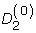is close to a compound Poisson. In the case of sequence larger than 1600 letters, the distribution ofis approximately normal. When estimating the significance of sequence similarity usingat its optimal word size, the null distribution would thus have to be adjusted according to the size of the sequences. It is worth noting that the size of typical ESTs or whole genome shotgun sequencing traces (500–800 letters) is in the transition region between these two limiting distributions.

We have also shown that the accuracy of thestatistic is similar to that of other similarity measures based on the number of words shared between two sequences. In particular, allowing for mismatches between words increases the accuracy of the. This improvement, however, comes at a high computation cost. The algorithmic complexity ofis o(kn), when t = 0, however, when t > 0, the worst case complexity is o(knm). In our simulations,was more accurate than BLAST (see figure 2). We expect this difference to be accentuated on real sequences where shuffling and transposition occurs, breaking the contiguity generally assumed by alignment-based sequence comparison algorithms.

The assumption of i.i.d. distributed letters made to ease the characterization of thedistribution may not hold in real sequences. This may have an impact on the assessment of the statistical significance of the number of words shared between two sequences. The optimal word sizes computed in this study, however, seem to be valid for real sequences evolved under a relatively realistic model of nucleotides substitution.

Taken together, the statistical theory, the algorithmic complexity and the simulation results suggest that the best application of thestatistics would be the identification of similarities between large genomic sequences using exact word matches. Comparison of such sequences using alignment-based methods is computationally expensive, since these algorithms typically have a o(n2) complexity, hence the development of heuristics speeding up these comparisons, such as BLAT  or MEGABLAST . The linear (o(n)) nature of thealgorithm and the normal asymptotic behavior of, when sequences are large compared to word size, would allow sequence similarity to be assessed in a rigorous statistical framework, with significant improvement in run time.

Finally, the known asymptotic behavior of thestatistic could be used to improve the assessment of significant matches during the initial (search) stage of existing alignment algorithms such as BLAST or BLAT.

The use ofin the context of biological sequences is not limited to the sequence comparison discussed in this paper. It has been proposed that it could be used to choose discriminative microarray probes . Other possible applications may include the detection of transcription factor binding sites, microRNAs and dsRNA targets.

## Methods

### Word size optimization

The optimization of the word size, for a given sequence length and number of mismatches, was carried out according to a method similar to that introduced in . In brief, a family of sequences was generated by first creating a random mother sequence. 100 sons were then derived by mutating γ% of the mother sequence, where γ = 1, 2, . . ., 100%. Only point mutations were used: substitution, insertion and deletion of a single letter. Thestatistic between the mother and each son were computed. Two rankings of the sons were then produced, one based on, and another based on γ. The accuracy of-based sequence comparison was estimated by looking at the discrepancy between these two rankings by means of the Spearman's rank statistic A. The optimal word size is that for which A is minimal. For each condition, the data presented here are the average of A for 100 to 400 families.

Similar simulations were also carried out by randomly selecting a mother sequence from the human genome chromosome 1, version NCBI36.40 (available from http://www.ensembl.org). Sons where then derived according to a K80  model of evolution.

A program written in ANSI C was written to compute the spearmanRS statistic. Data were post-processed using the R environment. The simulations were carried out on a Debian Gnu/Linux desktop computer. The source code is available from our k-words website .

### Fit to the normal distribution

Kolmogorov-Smirnov p-values  for the standardized statistic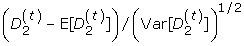compared with the standard normal distribution for sample sizes of 2,500 sequence pairs were computed. The results are shown in figure 1. In general, samples which are a close approximation to the normal distribution have p-values distributed uniformly in the interval [0, 1], whereas samples which are a poor approximation have small p-values.

## References

1. 1.

Altschul SF, Madden TL, Schaffer AA, Zhang J, Zhang Z, Miller W, Lipman DJ: Gapped BLAST and PSI-BLAST: a new generation of protein database search programs. Nucleic Acids Res 1997, 25(17):3389–402. 10.1093/nar/25.17.3389

2. 2.

Pearson WR: Rapid and sensitive sequence comparison with FASTP and FASTA. Methods Enzymol 1990, 183: 63–98.

3. 3.

Kent WJ: BLAT-the BLAST-like alignment tool. Genome Res 2002, 12(4):656–64. 10.1101/gr.229202. Article published online before March 2002

4. 4.

Florea L, Hartzell G, Zhang Z, Rubin GM, Miller W: A computer program for aligning a cDNA sequence with a genomic DNA sequence. Genome Res 1998, 8(9):967–74.

5. 5.

Vinga S, Almeida J: Alignment-free sequence comparison-a review. Bioinformatics 2003, 19(4):513–23. 10.1093/bioinformatics/btg005

6. 6.

Hide W, Burke J, Davison DB: Biological evaluation of d2, an algorithm for high-performance sequence comparison. J Comput Biol 1994, 1(3):199–215.

7. 7.

Burke J, Davison D, Hide W: d2_cluster: a validated method for clustering EST and full-length cDNAsequences. Genome Res 1999, 9(11):1135–42. 10.1101/gr.9.11.1135

8. 8.

Christoffels A, van Gelder A, Greyling G, Miller R, Hide T, Hide W: STACK: Sequence Tag Alignment and Consensus Knowledgebase. Nucleic Acids Res 2001, 29: 234–8. 10.1093/nar/29.1.234

9. 9.

Carpenter JE, Christoffels A, Weinbach Y, Hide WA: Assessment of the parallelization approach of d2 cluster for high-performance sequence clustering. J Comput Chem 2002, 23(7):755–7. 10.1002/jcc.10025

10. 10.

Lippert RA, Huang H, Waterman MS: Distributional regimes for the number of k-word matches between two random sequences. Proc Natl Acad Sci U S A 2002, 99(22):13980–9. 10.1073/pnas.202468099

11. 11.

Kantorovitz MR, Booth HS, Burden CJ, Wilson SR: Asymptotic behavior of k-word matches between two uniformly distributed sequences. preprint 2006.

12. 12.

Melko OM, Mushegian AR: Distribution of words with a predefined range of mismatches to a DNA probe in bacterial genomes. Bioinformatics 2004, 20: 67–74. 10.1093/bioinformatics/btg374

13. 13.

Burden CJ, Kantorovitz MR, Wilson SR: Approximate word matches between two random sequences. preprint 2006.

14. 14.

Waterman MS: Introduction to Computational Biology. Chapman and Hall; 1995.

15. 15.

Barbour A, Chryssaphinou O: Compound Poisson approximation: a user guide. Annals of Applied Probability 2001, 11(3):964–1002. 10.1214/aoap/1015345355

16. 16.

Wu TJ, Huang YH, Li LA: Optimal word sizes for dissimilarity measures and estimation of the degree of dissimilarity between DNA sequences. Bioinformatics 2005, 21(22):4125–32. 10.1093/bioinformatics/bti658

17. 17.

Kimura M: A simple method for estimating evolutionary rates of base substitutions through comparative studies of nucleotide sequences. J Mol Evol 1980, 16(2):111–20. 10.1007/BF01731581

18. 18.

Zhang Z, Schwartz S, Wagner L, Miller W: A greedy algorithm for aligning DNA sequences. J Comput Biol 2000, 7(1–2):203–14. 10.1089/10665270050081478

19. 19.

Source code for k-words[http://dayhoff.anu.edu.au/~sf/k_words.]

20. 20.

Conover WJ: Practical Nonparametric Statistics. John Wiley and Sons; 1999.

Download references

## Acknowledgements

This work was funded in part by ARC Discovery Grant DP0559260.

This article has been published as part of BMC Bioinformatics Volume 7, Supplement 5, 2006: APBioNet – Fifth International Conference on Bioinformatics (InCoB2006). The full contents of the supplement are available online at http://www.biomedcentral.com/1471-2105/7?issue=S5.

## Author information

Authors

### Corresponding author

Correspondence to Sylvain Forêt.

## Additional information

### Authors contributions

SF drafted most of the manuscript and performed the numerical simulations. MK made all the theoretical calculations. CB conceived of and coordinated the project and drafted parts of the manuscript. All authors read and approved the final manuscript.

## Electronic supplementary material

### 12859_2006_1371_MOESM3_ESM.eps

Additional file 3: Word size optimization for approximate word matches with 1 mismatch, non-uniform sequences (EPS 13 KB)

### 12859_2006_1371_MOESM4_ESM.eps

Additional file 4: Word size optimization for approximate word matches with 2 mismatch, non-uniform sequences (EPS 14 KB)

### 12859_2006_1371_MOESM6_ESM.eps

Additional file 6: Word size optimization for approximate word matches with 3 mismatch, non-uniform sequences (EPS 15 KB)

### 12859_2006_1371_MOESM7_ESM.eps

Additional file 7: Word size optimization for approximate word matches with 4 mismatch, non-uniform sequences (EPS 14 KB)

### 12859_2006_1371_MOESM8_ESM.eps

Additional file 8: Word size optimization for approximate word matches with 5 mismatch, non-uniform sequences (EPS 14 KB)

### 12859_2006_1371_MOESM9_ESM.eps

Additional file 9: Word size optimization for exact and approximate word matches, sequences evolving according to a Kimura80 substitution model. The dotted lines show the results obtained with blast. (EPS 20 KB)

## Rights and permissions

Open Access This article is published under license to BioMed Central Ltd. This is an Open Access article is distributed under the terms of the Creative Commons Attribution 2.0 International License (https://creativecommons.org/licenses/by/2.0), which permits unrestricted use, distribution, and reproduction in any medium, provided the original work is properly cited.

Reprints and Permissions

## About this article

### Cite this article

Forêt, S., Kantorovitz, M.R. & Burden, C.J. Asymptotic behaviour and optimal word size for exact and approximate word matches between random sequences. BMC Bioinformatics 7, S21 (2006). https://doi.org/10.1186/1471-2105-7-S5-S21

Download citation

• Published:

### Keywords

• Genome Shuffling
• Word Size
• Word Match
• Approximate Match
• Count Vector